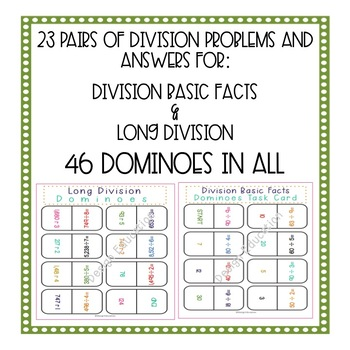# Division Game DominoesSubject
Resource Type
File Type

PDF

(3 MB|29 pages)
Product Rating
Standards
Also included in:
1. This bundle of domino games are the perfect way for students to practice the 4 operations. Each domino set includes two different versions of the game for easy differentiation. Meet all students needs in addition, subtraction, multiplication and division. What is included?Black and White printing
\$11.00
\$7.70
Save \$3.30
2. This bundle of domino games are the perfect way for students to practice key math concepts. Games included are addition, subtraction, multiplication, division, telling time, order of operations, finding place value in whole numbers, area & perimeter, geometry, math vocabulary, mixed and imprope
\$26.50
\$18.55
Save \$7.95
• Product Description
• StandardsNEW

Are you looking for a fun way for your students to practice division? This is it! Division Dominoes are awesome to incorporate into math centers, use for early finishers, or play on a math game day. This is an engaging way to practice foundational math skills. There are 2 versions for easy differentiation Basic Facts Division and Long Division.

What's Included?

• Black and White printing option
• Color printing option
• 2 Games each with 24 dominoes
• An answer key for each game so students can self-correct
• Student Recording Sheet
• Teacher Directions

Checkout my Other Domino Products:

Multiples and Factors

Rounding

Counting Coins

Find all factor pairs for a whole number in the range 1-100. Recognize that a whole number is a multiple of each of its factors. Determine whether a given whole number in the range 1-100 is a multiple of a given one-digit number. Determine whether a given whole number in the range 1-100 is prime or composite.
Multiply or divide to solve word problems involving multiplicative comparison, e.g., by using drawings and equations with a symbol for the unknown number to represent the problem, distinguishing multiplicative comparison from additive comparison.
Interpret a multiplication equation as a comparison, e.g., interpret 35 = 5 × 7 as a statement that 35 is 5 times as many as 7 and 7 times as many as 5. Represent verbal statements of multiplicative comparisons as multiplication equations.
Fluently multiply and divide within 100, using strategies such as the relationship between multiplication and division (e.g., knowing that 8 × 5 = 40, one knows 40 ÷ 5 = 8) or properties of operations. By the end of Grade 3, know from memory all products of two one-digit numbers.
Understand division as an unknown-factor problem. For example, find 32 ÷ 8 by finding the number that makes 32 when multiplied by 8.
Total Pages
29 pages
Included
Teaching Duration
N/A
Report this Resource to TpT
Reported resources will be reviewed by our team. Report this resource to let us know if this resource violates TpT’s content guidelines.